Courses

# Coupling Constants - Spectroscopy Chemistry Notes | EduRev

## Chemistry : Coupling Constants - Spectroscopy Chemistry Notes | EduRev

The document Coupling Constants - Spectroscopy Chemistry Notes | EduRev is a part of the Chemistry Course Organic Chemistry.
All you need of Chemistry at this link: Chemistry

Coupling Constant

Coupling constant is a quantitative measure of spin-spin splitting. It denotes how strongly a nucleus is affected by the spin-state of its neighbour.

• It is Distance between the peaks of multiplet
• Measured in Hz
• Not dependent on strength of the external field
• Multiplets with the same coupling constants may come from adjacent groups of protons that split each other.
• Coupling constant is a constant. It does not change in magnitude with change in instrument frequency.
• Increase in instrument frequency increases only the chemical shift of the nuclei, which increases the resolution of the spectrum.
• J can be either positive or negative, but only the absolute value is considered.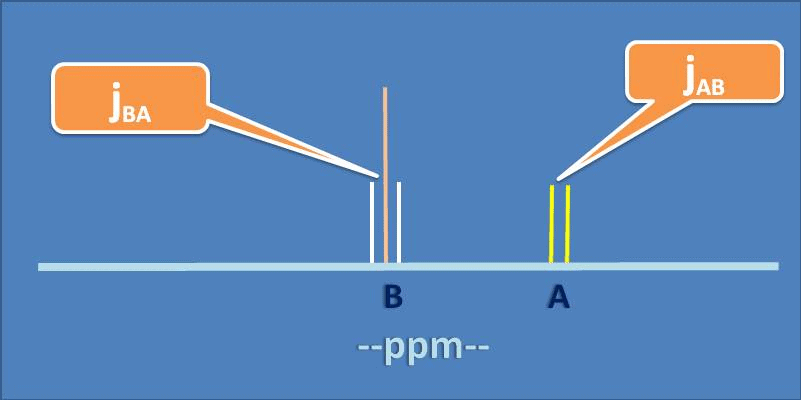Types of Coupling Constant

1. Based on the coupling partner:

• Homonuclear Coupling: Coupling between two nuclei of the same atom.
• Heteronuclear Coupling: Coupling between two nuclei of different atoms.

2. Based on the number of intervening bonds:

• One Bond Coupling (1J): Strong coupling, mainly heteronuclear.
• Two Bond Coupling (2J): Geminal coupling, high in magnitude.
• Three Bond Coupling (3J): Vicinal coupling, lower in magnitude, but highly significant for analysis.

3. Others: Specific couplings of higher order can be extremely usefulin NMR analysis.

• Ex: meta coupling, allylic coupling, W-coupling.

One Bond (1J) Coupling

Adjacent nuclei prefer to be in opposite spin states in the ground state. For a typical C—H bond, two peaks are observed in the 13C spectrum, due to the two shown transitions.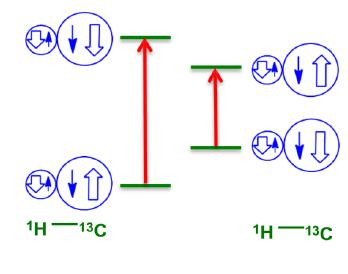Some common one-bond coupling constants have been displayed below: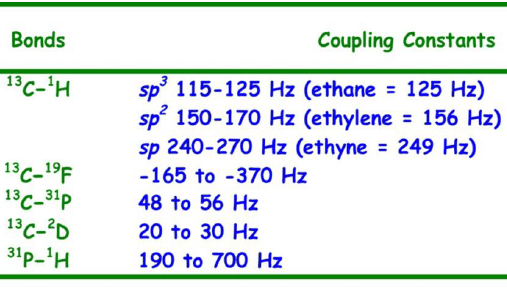Two Bond (2J) Coupling

Commonly called as geminal coupling, these are usually smaller in magnitude than 1 bond coupling.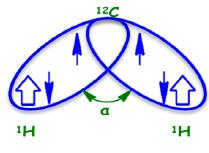Some common 2 bond coupling constants: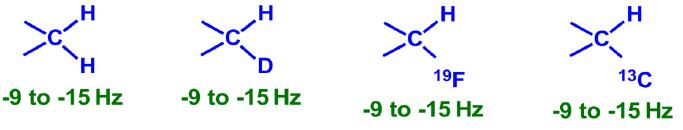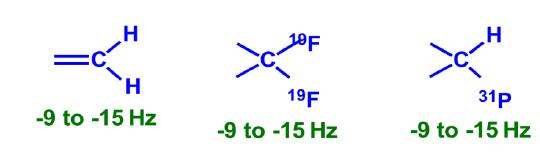Variation in 2J Coupling

Geminal coupling increases in magnitude as the decreases, due to higher electron spin correlation.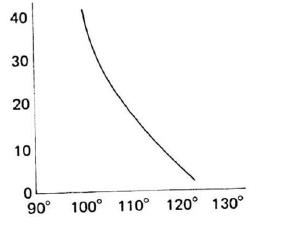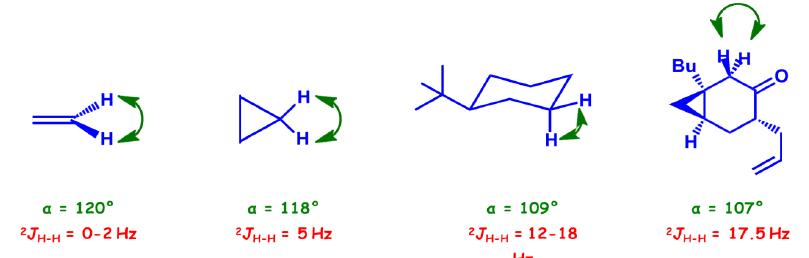Three Bond (3J) Coupling

Commonly called vicinal coupling, these usually follow the (n+1) rule in simple aliphatic hydrocarbon chains. Nuclear and electronic spin interactions carry the spin information from one hydrogen to its neighbor.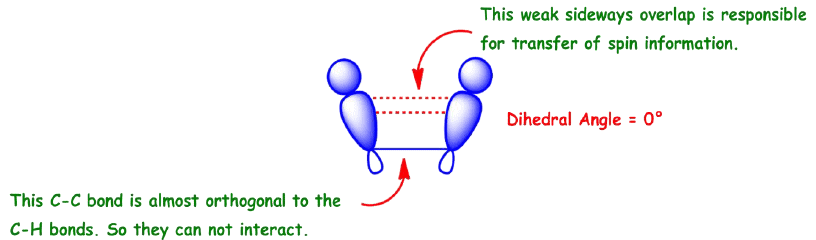As a result, the best overlap occurs when the C—H bonds are at a dihedral angle of 0°.

Variation in 3J Coupling

The magnitude of vicinal coupling is directly related to the dihedral angle between the C—H bonds in question. The Karplus equation relates dihedral angle to the coupling constant between two vicinal hydrogen nuclei.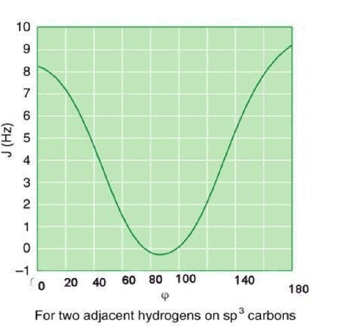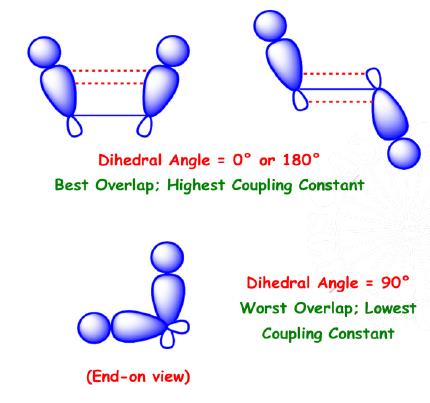Coupling Constant of cis/trans alkenes: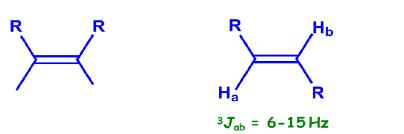Coupling Constant of Cyclohexane systems: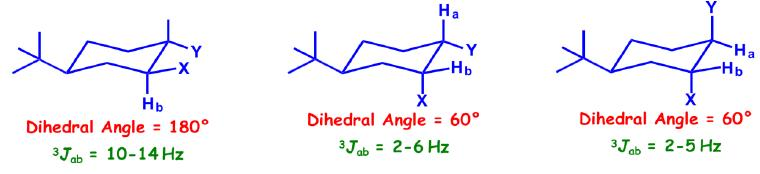Coupling Constant of Cyclopropane/Epoxide Systems:

Jcis > Jtrans (unlike in alkenes)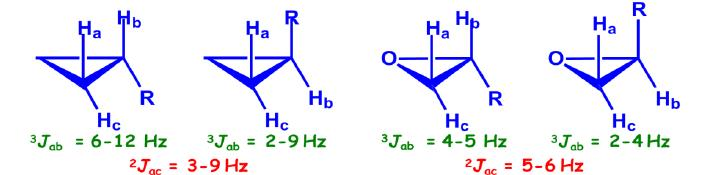Long-Range Coupling

Very rarely, but often significantly, coupling takes place between two atoms separated by four bonds or more (nJ; n≥4). The common systems where this is exhibited are allylic systems, rigid bicyclic systems, and aromatic rings. Since these couplings are observed through a large number of bonds, a highly specific stereochemical arrangement is essential.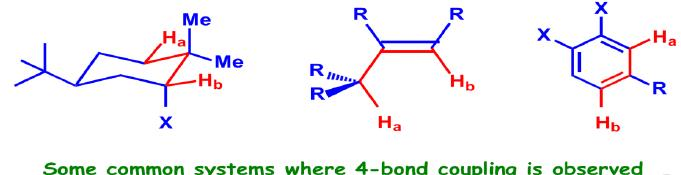Stereochemical Nonequivalence:

• Usually, two protons on the same C are equivalent and do not split each other.
• If the replacement of each of the protons of a —CH2 group with an imaginary “Z” gives stereoisomers, then the protons are non-equivalent and will split each other.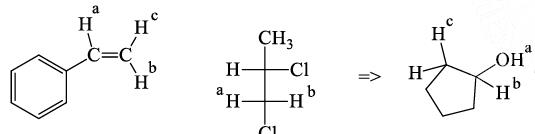Offer running on EduRev: Apply code STAYHOME200 to get INR 200 off on our premium plan EduRev Infinity!

## Organic Chemistry

39 videos|71 docs|34 tests

,

,

,

,

,

,

,

,

,

,

,

,

,

,

,

,

,

,

,

,

,

;